# PSAT Math : Equations / Inequalities

## Example Questions

← Previous 1 3 4 5 6 7 8 9 17 18

### Example Question #1 : Equations / Inequalities

Factor 4x3 – 16x

4x(x – 2)(x – 2)

4x(x + 2)(x + 2)

cannot be factored

4x(x + 2)(x – 2)

4x(x2 – 4)

4x(x + 2)(x – 2)

Explanation:

First pull out any common terms: 4x3 – 16x = 4x(x2 – 4)

x2 – 4 is a difference of squares, so we can also factor that further. The difference of squares formula is a2 – b2 = (a – b)(a + b). Here a = x and b = 2. So x2 – 4 = (x – 2)(x + 2).

Putting everything together, 4x3 – 16x = 4x(+ 2)(– 2).

### Example Question #1 : Equations / Solution Sets

Factor the following equation.

x– 16

(x2)(4 – 2)

(x)(x – 4)

(x + 4)(x – 4)

(x – 4)(x – 4)

(x + 4)(x + 4)

(x + 4)(x – 4)

Explanation:

The correct answer is (x + 4)(x – 4)

We neen to factor x– 16 to solve. We know that each parenthesis will contain an x to make the x2. We know that the root of 16 is 4 and since it is negative and no value of x is present we can tell that one 4 must be positive and the other negative. If we work it from the multiple choice answers we will see that when multiplying it out we get x+ 4x – 4x – 16. 4x – 4x cancels out and we are left with our answer.

### Example Question #2 : Equations / Inequalities

If x3 – y3 = 30, and x2 + xy + y2 = 6, then what is x2 – 2xy + y2?

24

cannot be determined

180

5

25

25

Explanation:

First, let's factor x3 – y3 using the formula for difference of cubes.

x3 – y= (x – y)(x2 + xy + y2)

We are told that x2 + xy + y2 = 6. Thus, we can substitute 6 into the above equation and solve for x – y.

(x - y)(6) = 30.

Divide both sides by 6.

x – y = 5.

The original questions asks us to find x2 – 2xy + y2. Notice that if we factor x2 – 2xy + y2 using the formula for perfect squares, we obtain the following:

x2 – 2xy + y= (x – y)2.

Since we know that (x – y) = 5, (x – y)2 must equal 52, or 25.

Thus, x2 – 2xy + y= 25.

### Example Question #2 : Equations / Inequalities

if x – y = 4 and x2 – y = 34, what is x?

6

10

9

12

15

6

Explanation:

This can be solved by substitution and factoring.

x2 – y = 34 can be written as y = x2 – 34 and substituted into the other equation: x – y = 4 which leads to x – x2 + 34 = 4 which can be written as x2 – x – 30 = 0.

x2 – x – 30 = 0 can be factored to (x – 6)(x + 5) = 0 so x = 6 and –5 and because only 6 is a possible answer, it is the correct choice.

### Example Question #2 : Equations / Inequalities

If x2 + 2ax + 81 = 0.  When a = 9, what is the value of x?

9

–9

–18

0

3

–9

Explanation:

When a = 9, then x2 + 2ax + 81 = 0 becomes

x2 + 18x + 81 = 0.

This equation can be factored as (x + 9)2 = 0.

Therefore when a = 9, x = –9.

### Example Question #2 : How To Factor An Equation

If f(x) has roots at x = –1, 0 and 2, which of the following could be the equation for f(x)?

f(x) = x4 + x3 – 2x2

f(x) = x– x– 2x

f(x) = x2 + x – 2

f(x) = x– x+ 2x

f(x) = x– x – 2

f(x) = x– x– 2x

Explanation:

In general, if a function has a root at x = r, then (x – r) must be a factor of f(x). In this problem, we are told that f(x) has roots at –1, 0 and 2. This means that the following are all factors of f(x):

(x – (–1)) = x + 1

(x – 0) = x

and (x – 2).

This means that we must look for an equation for f(x) that has the factors (+ 1), x, and (x – 2).

We can immediately eliminate the function f(x) = x2 + x – 2, because we cannot factor an x out of this polynomial. For the same reason, we can eliminate f(x) = x– x – 2.

Let's look at the function f(x) = x– x+ 2x. When we factor this, we are left with x(x2 – x + 2). We cannot factor this polynomial any further. Thus, x + 1 and x – 2 are not factors of this function, so it can't be the answer.

Next, let's examine f(x) = x4 + x3 – 2x.

We can factor out x2.

x(x+ x – 2)

When we factor x+ x – 2, we will get (x + 2)(x – 1). These factors are not the same as x – 2 and x + 1.

The only function with the right factors is f(x) = x– x– 2x.

When we factor out an x, we get (x– x – 2), which then factors into (x – 2)(+ 1). Thus, this function has all of the factors we need.

The answer is f(x) = x– x– 2x.

### Example Question #1 : Factoring Equations

Factor 36x2 – 49y2.

(6x – 7y)(6x – 7y)

(6x + 7y)(6x + 7y)

6x2 – 7y2

(6x + 7y)(6x – 7y)

cannot be factored

(6x + 7y)(6x – 7y)

Explanation:

This is a difference of squares. The difference of squares formula is a2 – b2 = (a + b)(a – b). In this problem, a = 6x and b = 7y.

So 36x2 – 49y= (6x + 7y)(6x – 7y).

### Example Question #7 : How To Factor An Equation

Solve for x: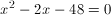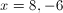Explanation:

Find two numbers that add to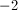and multiply to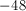Factors of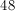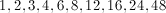You can use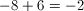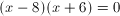Then make each factor equal 0.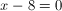and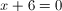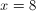and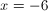### Example Question #1 : How To Factor An Equation

Find the roots of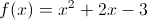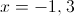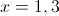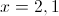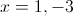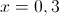Explanation:

Factoring yields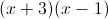giving roots of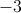and.

### Example Question #2 : Factoring Equations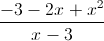Find the root of the equation above.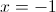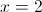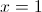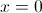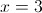Explanation:

The numerator can be factored into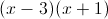.

Therefore, it can cancel with the denominator. So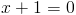imples.

← Previous 1 3 4 5 6 7 8 9 17 18

### All PSAT Math Resources